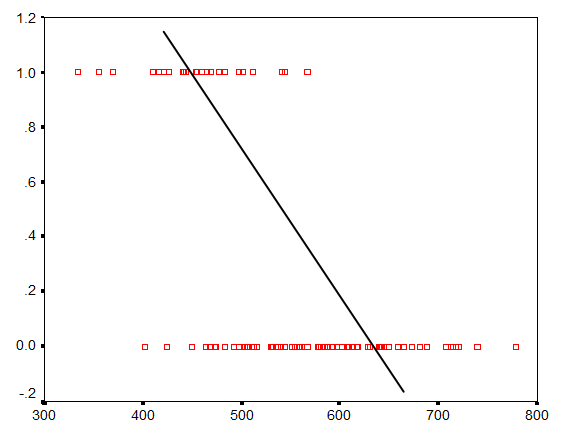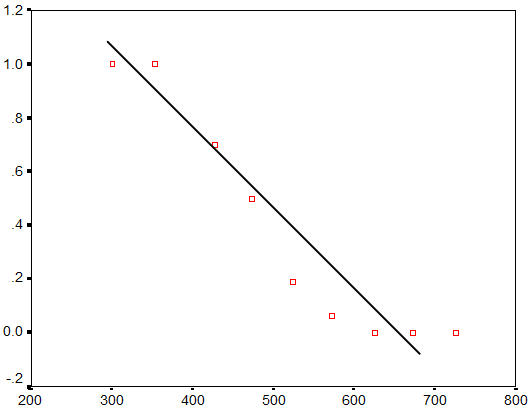# What is a Logit Function and Why Use Logistic Regression?

One of the big assumptions of linear models is that the residuals are normally distributed.  This doesn’t mean that Y, the response variable, has to also be normally distributed, but it does have to be continuous, unbounded and measured on an interval or ratio scale.

Unfortunately, categorical response variables are none of these.

No matter how many transformations you try, you’re just never going to get normal residuals from a model with a categorical response variable.

There are a number of alternatives though, and one of the most popular is logistic regression.

In many ways, logistic regression is very similar to linear regression.  One big difference, though, is the logit link function.

A link function is simply a function of the mean of the response variable Y that we use as the response instead of Y itself.

All that means is when Y is categorical, we use the logit of Y as the response in our regression equation instead of just Y:The logit function is the natural log of the odds that Y equals one of the categories.  For mathematical simplicity, we’re going to assume Y has only two categories and code them as 0 and 1.

This is entirely arbitrary–we could have used any numbers.  But these make the math work out nicely, so let’s stick with them.

P is defined as the probability that Y=1.  So for example, those Xs could be specific risk factors, like age, high blood pressure, and cholesterol level, and P would be the probability that a patient develops heart disease.

### Why Bother With This Logit Function?

Well, if we used Y as the outcome variable and tried to fit a line, it wouldn’t be a very good representation of the relationship.  The following graph shows an attempt to fit a line between one X variable and a binary outcome Y.

You can see a relationship there–higher values of X are associated with more 0s and lower values of X have more 1s.  But it’s not a linear relationship.Okay, fine.  But why mess with logs and odds?  Why not just use P as the outcome variable?  Everyone understands probability.

Here’s the same graph with probability on the Y axis:It’s closer to being linear, but it’s still not quite there.  Instead of a linear relationship between X and P, we have a sigmoidal or S-shaped relationship.

But it turns out that there are a few functions of P that do form reasonably linear relationships with X.  These include:

• Square root of arcsin
• Complimentary log-log
• Probit
• Logit

The logit function is particularly popular because, believe it or not, its results are  relatively easy to interpret.  But many of the others work just as well.

Once we fit this model, we can then back-transform the estimated regression coefficients off of a log scale so that we can interpret the conditional effects of each X.Binary, Ordinal, and Multinomial Logistic Regression for Categorical Outcomes
Get beyond the frustration of learning odds ratios, logit link functions, and proportional odds assumptions on your own. See the incredible usefulness of logistic regression and categorical data analysis in this one-hour training.

1.Shahab Hadaegh says

Given the non-linearity of the transformation, can back-transforming the estimated coefficients result in bias ?

2.Lisa says

Hello,
what’s the difference between performing a logistic regression and performing a linear regression based on a [previously computed] logit as dependent variable? Many thanks in advance.

•Karen Grace-Martin says

Hi Lisa,

A logit as dependent variable doesn’t really work when the outcome is 1/0. You’d have to group observations to come up with a value of p in the logit–the proportion of 1s. That’s the beauty of the link function. It does that for you.

3.afvrefz says

Karen,

Can you list all possible functions used in GRL please ?

Example: logictic function, square root of arcsin,…

Thank you

4.may says

How can i used Square root of arcsin as a model in logistic regression for binomial data?

•Karen Grace-Martin says

May, you can’t. Square root of arcsin is an alternative to logistic regression, but it’s arcane. It is still recommended sometimes, but it’s an ad-hoc way of fitting a binary outcome into a normal model. It’s better to just do the logistic regression.

5.faisal says

Aoa, please tell me that will i use probit o logit model if, i have one dependent and one independent model with five control variables. dependent variable is a dummy variable 0 and 1 and also 1 control variable have values 1 and 0??

•Karen Grace-Martin says

In that situation, you should be able to use either probit or logistic.

6.Hamedi says

In GLM models, Is it possible to use a function of median instead of a function of the mean of the response in the logit link? I would use a distribution which it’s median is simpler than mean. Could I use median instead of mean?

•Karen Grace-Martin says

Hi Hamedi,

Not that I’ve ever heard. The median of a binary outcome would be either 0 or 1. The mean is somewhere in between – it’s the proportion of 1s.

7.Tom says

What will be the form of the logit function in case that Y is a binary variable such that y=0 with probability p and y=1 with probability (1-p).
Thanks

•Karen Grace-Martin says

Hi Tom,

The form will be the same. You’re just switching what is a success (Y=1) with what is a failure (Y=0).

8.V. Mahdavi says

Is it possible negative slope of the line in the chart probit؟

9.Raj says

Hello,
I am some doubt regarding adding interaction term in a model. When we add interaction term in a model and how to interpret the coefficient of interaction. If you have some paper or book, kindly send it to me. It helps me a lot.
Thanks

•Karen says

Hi Raj,

I’m not sure I can think of anything written on interpreting interactions in logistic regression, but we do cover this in the logistic regression workshop.

The very basic idea, though, is that the odds ratio for an interaction is the ratio of odds ratios. It’s hard to explain without knowing if the terms you are interacting are continuous or categorical, but that’s the basic definition. It takes me a good half hour to go over this in the workshop. 🙂

•Bruce Weaver says

Hello Raj. You may find this book helpful:

Jaccard, J., & Turrisi, R. (2003). Interaction effects in multiple regression (No. 72). Sage.

As it explains, the coefficient for a product term shows a difference in differences of logits. And therefore, Exp(B) for the product term shows a ratio of odds ratios.

Cheers,
Bruce Weaver

Please note that, due to the large number of comments submitted, any questions on problems related to a personal study/project will not be answered. We suggest joining Statistically Speaking, where you have access to a private forum and more resources 24/7.